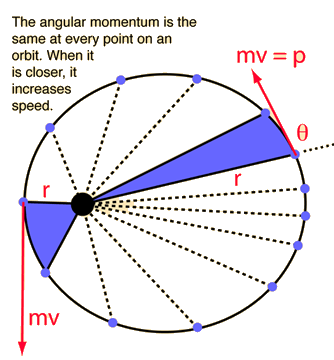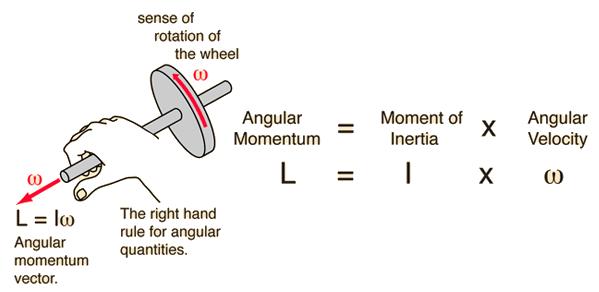# Angular Momentum of a ParticleThe angular momentum of a particle of mass m with respect to a chosen origin is given by L = mvr sin θ or more formally by the vector product L = r x p The direction is given by the right hand rule which would give L the direction out of the diagram. For an orbit, angular momentum is conserved, and this leads to one of Kepler's laws. For a circular orbit, L becomes L = mvr
Index

Angular momentum of rigid body

 HyperPhysics***** Mechanics *****Rotational motion R Nave
Go Back

# Angular Momentum

The angular momentum of a rigid object is defined as the product of the moment of inertia and the angular velocity. It is analogous to linear momentum and is subject to the fundamental constraints of the conservation of angular momentum principle if there is no external torque on the object. Angular momentum is a vector quantity. It is derivable from the expression for the angular momentum of a particleComparison of linear and angular momentum
Index

Moment of inertia concepts

 HyperPhysics***** Mechanics *****Rotational motion R Nave
Go Back

# Angular and Linear Momentum

Angular momentum and linear momentum are examples of the parallels between linear and rotational motion. They have the same form and are subject to the fundamental constraints of conservation laws, the conservation of momentum and the conservation of angular momentum .Index

Moment of inertia concepts

 HyperPhysics***** Mechanics *****Rotational motion R Nave
Go Back